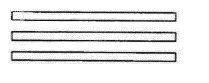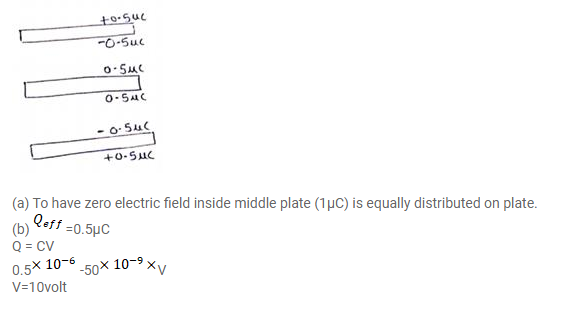# The capacitance between the adjacent plates shown in figure isQuestion:

The capacitance between the adjacent plates shown in figure is $50 \mathrm{nF}$. A charge of I.0 $\mu \mathrm{C}$ is placed on the middle plate,

(a) What will be the charge on the outer surface of the upper plate?

(b) Find the potential difference developed between the upper and the middle plates.Solution: